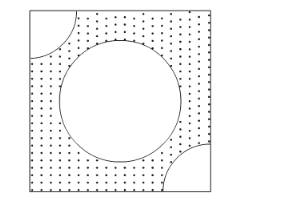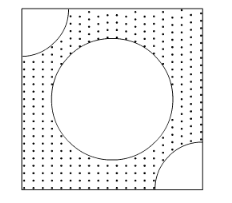# From each of the two opposite corners of a square of side 8 cm,Question:

From each of the two opposite corners of a square of side 8 cm, a quadrant of a circle of radius 1.4 cm is cut. Another circle of radius 4.2 cm is also cut from the centre as shown in the following figure. Find the area of the remaining (Shaded) portion of the square. (Use π = 22/7)Solution:

It is given that a circle of radius 4.2 cm and two quadrants of radius 1.4 cm are cut from a square of side 8 cm.Let the side of square be a. Then,

Area of square $=a^{2}$

$=8 \times 8$

$=64 \mathrm{~cm}^{2}$

Since the radius of circle is 4.2 cm. So,

Area of circle $=\pi \mathrm{r}^{2}$

$=\frac{22}{7} \times 4.2 \times 4.2$

$=55.44 \mathrm{~cm}^{2}$

Area of quadrant $=\frac{1}{4} \pi r^{2}$

$=\frac{1}{4} \times \frac{22}{7} \times 1.4 \times 1.4$

$=1.54 \mathrm{~cm}^{2}$

Area of shaded region $=$ Area of square - Area of circle $-2 \times$ Area of quadrant

$=64-55.44-2 \times 1.54$

$=5.48 \mathrm{~cm}^{2}$